40 斐波那契数列## 什么是斐波那契数列？

### 理解递归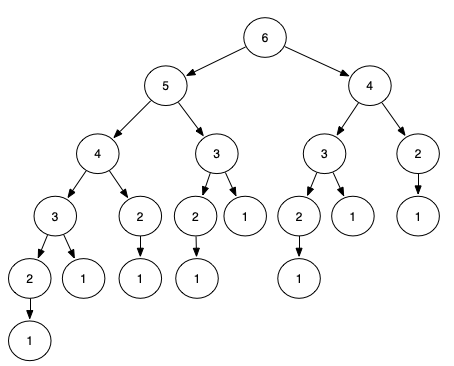### 记忆化搜索

int cache[N]; //n如果有确切的范围，并且n比较小时，我们可以开个数组来存储函数值，存取函数值只有o(1)的复杂度
// map<int, int> cache; //如果没有确切的范围，或者n比较大时，我们需要利用映射数据结构来存储函数值，比如红黑树、哈希表
memset(cache, -1, sizeof(cache)); //-1表示cache还没计算过
int f(int n) {
if (n <= 2) {
return 1;
}
if (cache[n] != -1) {
return cache[n];
}
return cache[n] = f(n - 1) + f(n - 2);
}

### 问题四

n的数据范围超级大，达到10^18，已经无法用记忆化搜索或者递推来做，这就需要另一种做法。

### 快速幂算法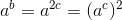，我们只需要求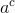，就可以以o(1)的时间复杂度得到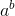，我们同样只需要求，就可以以o(1)的时间复杂度得到int f(int a, int b, int k) {
if  (b == 1)  { return a % k; }
//求解a ^ (b / 2)
int ret = f(a, b >> 1, k);
//求解 (a ^ (b / 2)) ^ 2
ret = (long long) ret * ret % k;
if (b & 1) {
//处理b是奇数的情况，多乘了一次a
ret = (long long) ret *a % k;
}
return ret;
}

### 斐波那契数列的快速幂算法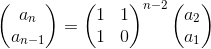Matrix mod(Matrix& A, int k);
Matrix multiply(Matrix& A, Matrix& B, int k);
int pow(Matrix& A, long long b, int k) {
if  (b == 1)  { return mod(A, k); }
Matrix ret = pow(a, b >> 1, k);
ret = multiply(ret, ret, k);
if (b & 1) {
ret = multiply(ret, a, k);
}
return ret;
}

### 拓展 Fibonacci 的DP版本

• top-down(memorize)：
def memorize_fib(n): # n为第几个Fibonacci数
memo = {1:1, 2:1}
if n in memo:
return memo[n]
else:
memo[n] = memorize_fib(n-1) + memorize_fib(n-2)
return memo[n]

print(memorize_fib(4)) # 输出3
• bottom up(tabulation)：
def tabulation_fib(n):  # n为第几个Fibonacci数
fib = [1, 1, 2]
if n < 4:
return fib[n-1]
for k in range(3, n+1):
fib = fib + fib
fib, fib = fib, fib
return fib

print(tabulation_fib(4)) # 输出3

import functools

@functools.lru_cache(maxsize=None)
def fib(n):
if n <= 2:
return 1
else:
return fib(n - 1) + fib(n - 2)

print(fib(10)) # 55=

## 小结